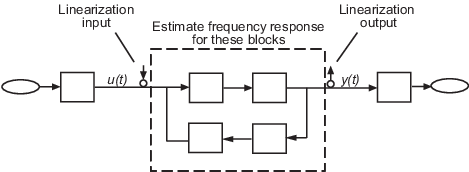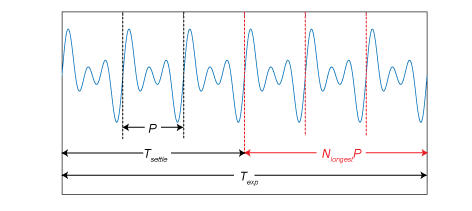Documentation

## Estimation Input Signals

Frequency response estimation requires an input signal to excite the model at frequencies of interest. The software then measures the response at the specified output, using the input signal and measured response to estimate the frequency response.When you perform frequency response estimation, you specify what type of input signal to use and what its properties are.

### Offline Estimation

The following table summarizes the types of input signals you can use for offline estimation in Linear Analysis Tool or at the MATLAB® command line for use with `frestimate`.

SignalDescription
Sinestream

A series of sinusoidal perturbations applied one after another. Sinestream signals are recommended for most situations. They are especially useful when your system contains strong nonlinearities or you require highly accurate frequency response models.

Chirp

A swept-frequency signal that excites your system at a range of frequencies, such that the input frequency changes instantaneously. Chirp signals are useful when your system is nearly linear in the simulation range. They are also useful when you want to obtain a response quickly for a lot of frequency points.

Random

A random input signal. Random signals are useful because they can excite the system uniformly at all frequencies up to the Nyquist frequency.

Step

A step input signal. Step inputs are quick to simulate and can be useful as a first try when you do not have much knowledge about the system you are trying to estimate.

Arbitrary

A MATLAB timeseries with which you can specify any time-varying signal as input.

In general, the estimated frequency response is related to the input and output signals as:

`$Resp=\frac{FFT\left({y}_{est}\left(t\right)\right)\text{\hspace{0.17em}}}{FFT\left({u}_{est}\left(t\right)\right)}.$`

Here, uest(t) is the injected input signal and yest (t) is the corresponding simulated output signal. For more details, see the Algorithms section of `frestimate`.

### Online Estimation

For online estimation with the Frequency Response Estimator block, you can use two types of input signals:

• Sinestream — A series of sinusoidal perturbations applied one after another

• Superposition — A set of sinusoidal perturbations applied simultaneously

For online estimation, using a sinestream signal can be more accurate and can accommodate a wider range of frequencies than a superposition signal. The sinestream mode can also be less intrusive. However, due to the sequential nature of the sinestream perturbation, each frequency point you add increases the experiment time. Thus the estimation experiment is typically much faster with a superposition signal with satisfactory results.

To specify which type of input signal to use for online estimation, use the Experiment mode parameter of the Frequency Response Estimator block.

### Sinestream Signals

For details about the structure of sinestream signals and how to create them, see Sinestream Input Signals.

### Chirp Signals

For details about the structure of chirp signals and how to create them, see Chirp Input Signals.

### Random Signals

Random signals are useful because they can excite the system uniformly at all frequencies up to the Nyquist frequency. To create a random input signal for estimation:

• In the Linear Analysis Tool, on the Estimation tab, select Input Signal > Random.

• At the command line, use `frest.Random` to create the random signal and use it as an input argument to `frestimate`.

The random signal comprises uniformly distributed random numbers in the interval `[0 Amplitude]` or `[Amplitude 0]` for positive and negative amplitudes, respectively. You can specify the amplitude, sample time, and number of samples directly when you create the input signal. Alternatively, if you have a relevant linear time-invariant (LTI) model such as a state-space (`ss`) model, you can use it to initialize the random signal parameters. For instance, if you have an exact linearization of your system, you can use it to initialize the parameters.

When you use a random input signal for estimation, the frequencies returned in the estimated `frd` model depend on the length and sampling time of the signal. They are the frequencies obtained in the fast Fourier transform of the input signal (see the Algorithm section of `frestimate`).

### Step Signals

Step inputs are quick to simulate. Like a random signal, a step signal can excite the system at all frequencies up to the Nyquist frequency. For those reasons, a step input can be useful as a first try when you do not have much knowledge about the system you are trying to estimate. However, the amplitude of the excitation decreases rapidly with increasing frequency. Therefore, step signals are best used to identify low-order plants where the slowest poles are dominant. Step inputs are not recommended for estimation across a wide range of frequencies.

To create a step input signal for estimation, use `frest.createStep`. This function creates a MATLAB `timeseries` that represents a step input having the sample time, step time, step size, and total signal length that you specify when you call `frest.createStep`.

To use the step input signal you created in the MATLAB workspace:

• In the Linear Analysis Tool, on the Estimation tab, select it from the Existing Input Signals section of the Input Signal drop-down list.

• At the command line, use it as an input argument to `frestimate`.

When you use a step input signal for estimation, the frequencies returned in the estimated `frd` model depend on the length and sampling time of the signal. They are the frequencies obtained in the fast Fourier transform of the input signal (see the Algorithm section of `frestimate`).

### Arbitrary Signals

If you want to use a signal other than a sinestream, chirp, step, or random signal, you can provide your own MATLAB `timeseries` object. For instance, you can create a `timeseries` representing a ramp, sawtooth, or square wave input.

To use a `timeseries` object as the input signal for estimation, first create the `timeseries` in the MATLAB workspace. Then:

• In the Linear Analysis Tool, on the Estimation tab, select it from the Existing Input Signals section of the Input Signal drop-down list.

• At the command line, use it as an input argument to `frestimate`.

When you use an arbitrary input signal for estimation, the frequencies returned in the estimated `frd` model depend on the length and sampling time of the signal. They are the frequencies obtained in the fast Fourier transform of the input signal (see the Algorithm section of `frestimate`).

### Superposition Signals

Superposition signals are available only for online estimation with the Frequency Response Estimator block. For frequency response estimation at a vector of frequencies ω = [ω1, … , ωN] at amplitudes A = [A1, … , AN], the superposition signal is given by:

`$\Delta u=\sum _{i}{A}_{i}\mathrm{sin}\left({\omega }_{i}t\right).$`

The block supplies the perturbation Δu for the duration of the experiment (while the start/stop signal is positive). The block determines how long to wait for system transients to die away and how many cycles to use for estimation as shown in the following illustration.Texp is the experiment duration that you specify with your configuration of the start/stop signal (See the start/stop port description on the block reference page for more information). For the estimation computation, the block uses only the data collected in a window of NlongestP. Here, P is the period of the slowest frequency in the frequency vector ω, and Nlongest is the value of the Number of periods of the lowest frequency used for estimation block parameter. Any cycles before this window are discarded. Thus, the settling time Tsettle = TexpNlongestP. If you know that your system settles quickly, you can shorten Texp without changing Nlongest to effectively shorten Tsettle. If your system is noisy, you can increase Nlongest to get more averaging in the data-collection window. Either way, always choose Texp long enough for sufficient settling and sufficient data-collection. The recommended Texp = 2NlongestP.

To use a superposition signal for estimation, in the Frequency Response Estimator block, set the Experiment mode parameter to Superposition. For details, see Frequency Response Estimator.